Chapter 17. Electrochemistry

# 17.5 Batteries and Fuel Cells

### Learning Objectives

By the end of this section, you will be able to:
• Classify batteries as primary or secondary
• List some of the characteristics and limitations of batteries
• Provide a general description of a fuel cell

A battery is an electrochemical cell or series of cells that produces an electric current. In principle, any galvanic cell could be used as a battery. An ideal battery would never run down, produce an unchanging voltage, and be capable of withstanding environmental extremes of heat and humidity. Real batteries strike a balance between ideal characteristics and practical limitations. For example, the mass of a car battery is about 18 kg or about 1% of the mass of an average car or light-duty truck. This type of battery would supply nearly unlimited energy if used in a smartphone, but would be rejected for this application because of its mass. Thus, no single battery is “best” and batteries are selected for a particular application, keeping things like the mass of the battery, its cost, reliability, and current capacity in mind. There are two basic types of batteries: primary and secondary. A few batteries of each type are described next.

# Primary Batteries

Primary batteries are single-use batteries because they cannot be recharged. A common primary battery is the dry cell (Figure 1). The dry cell is a zinc-carbon battery. The zinc can serves as both a container and the negative electrode. The positive electrode is a rod made of carbon that is surrounded by a paste of manganese(IV) oxide, zinc chloride, ammonium chloride, carbon powder, and a small amount of water. The reaction at the anode can be represented as the ordinary oxidation of zinc:

$\text{Zn}(s)\;{\longrightarrow}\;\text{Zn}^{2+}(aq)\;+\;2\text{e}^{-}\;\;\;\;\;\;\;E_{\text{Zn}^{2+}/\text{Zn}}^{\circ} = -0.7618\;\text{V}$

The reaction at the cathode is more complicated, in part because more than one reaction occurs. The series of reactions that occurs at the cathode is approximately

$2\text{MnO}_2(s)\;+\;2\text{NH}_4\text{Cl}(aq)\;+\;2\text{e}^{-}\;{\longrightarrow}\;\text{Mn}_2\text{O}_3(s)\;+\;2\text{NH}_3(aq)\;+\;\text{H}_2\text{O}(l)\;+\;2\text{Cl}^{-}$

The overall reaction for the zinc–carbon battery can be represented as $2\text{MnO}_2(s)\;+\;2\text{NH}_4\text{Cl}(aq)\;+\;\text{Zn}(s)\;{\longrightarrow}\;\text{Zn}^{2+}(aq)\;+\;\text{Mn}_2\text{O}_3(s)\;+\;2\text{NH}_3(aq)\;+\;\text{H}_2\text{O}(l)\;+\;2\text{Cl}^{-}$ with an overall cell potential which is initially about 1.5 V, but decreases as the battery is used. It is important to remember that the voltage delivered by a battery is the same regardless of the size of a battery. For this reason, D, C, A, AA, and AAA batteries all have the same voltage rating. However, larger batteries can deliver more moles of electrons. As the zinc container oxidizes, its contents eventually leak out, so this type of battery should not be left in any electrical device for extended periods.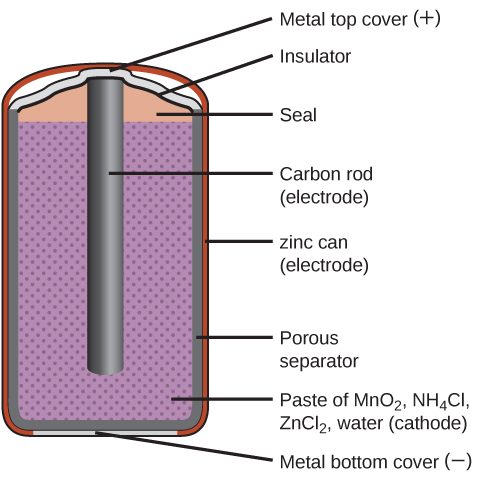Figure 1. The diagram shows a cross section of a flashlight battery, a zinc-carbon dry cell.

Alkaline batteries (Figure 2) were developed in the 1950s partly to address some of the performance issues with zinc–carbon dry cells. They are manufactured to be exact replacements for zinc-carbon dry cells. As their name suggests, these types of batteries use alkaline electrolytes, often potassium hydroxide. The reactions are

$\begin{array}{lr @{{}\longrightarrow{}} ll} \text{anode:} & \text{Zn}(s)\;+\;2\text{OH}^{-}(aq) & \text{ZnO}(s)\;+\;\text{H}_2\text{O}(l)\;+\;2\text{e}^{-} & E_{\text{anode}}^{\circ} = -1.28\;\text{V} \\[0.5em] \text{cathode:} & 2\text{MnO}_2(s)\;+\;\text{H}_2\text{O}(l)\;+\;2\text{e}^{-} & \text{Mn}_2\text{O}_3(s)\;+\;2\text{OH}^{-}(aq) & E_{\text{cathode}}^{\circ} = +0.15\;\text{V} \\[0.5em] \hline \\[-0.25em] \text{overall:} & \text{Zn}(s)\;+\;2\text{MnO}_2(s) & \text{ZnO}(s)\;+\;\text{Mn}_2\text{O}_3(s) & E_{\text{cell}}^{\circ} = +1.43\;\text{V} \end{array}$

An alkaline battery can deliver about three to five times the energy of a zinc-carbon dry cell of similar size. Alkaline batteries are prone to leaking potassium hydroxide, so these should also be removed from devices for long-term storage. While some alkaline batteries are rechargeable, most are not. Attempts to recharge an alkaline battery that is not rechargeable often leads to rupture of the battery and leakage of the potassium hydroxide electrolyte.

# Secondary Batteries

Secondary batteries are rechargeable. These are the types of batteries found in devices such as smartphones, electronic tablets, and automobiles.

Nickel-cadmium, or NiCd, batteries (Figure 3) consist of a nickel-plated cathode, cadmium-plated anode, and a potassium hydroxide electrode. The positive and negative plates, which are prevented from shorting by the separator, are rolled together and put into the case. This is a “jelly-roll” design and allows the NiCd cell to deliver much more current than a similar-sized alkaline battery. The reactions are

$\begin{array}{lr @{{}\longrightarrow{}} l} \text{anode:} & \text{Cd}(s)\;+\;2\text{OH}^{-}(aq) & \text{Cd(OH)}_2(s)\;+\;2\text{e}^{-} \\[0.5em] \text{cathode:} & \text{NiO}_2(s)\;+\;2\text{H}_2\text{O}(l)\;+\;2\text{e}^{-} & \text{Ni(OH)}_2(s)\;+\;2\text{OH}^{-}(aq) \\[0.5em] \hline \\[-0.25em] \text{overall:} & \text{Cd}(s)\;+\;\text{NiO}_2(s)\;+\;2\text{H}_2\text{O}(l) & \text{Cd(OH)}_2(s)\;+\;\text{Ni(OH)}_2(s) \end{array}$

The voltage is about 1.2 V to 1.25 V as the battery discharges. When properly treated, a NiCd battery can be recharged about 1000 times. Cadmium is a toxic heavy metal so NiCd batteries should never be opened or put into the regular trash.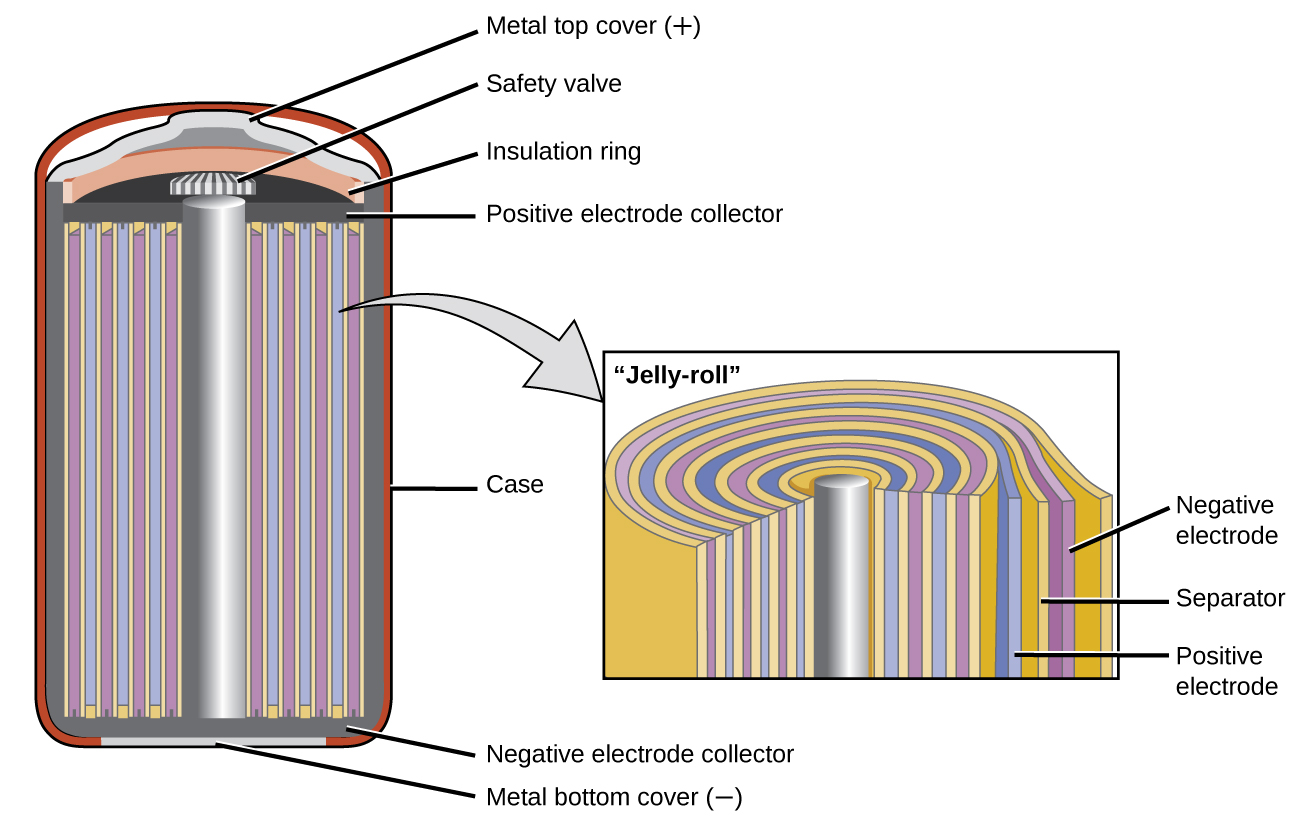Figure 3. NiCd batteries use a “jelly-roll” design that significantly increases the amount of current the battery can deliver as compared to a similar-sized alkaline battery.

Lithium ion batteries (Figure 4) are among the most popular rechargeable batteries and are used in many portable electronic devices. The reactions are

$\begin{array}{lr @{{}\leftrightharpoons{}} l} \text{anode:} & \text{LiCoO}_2 & \text{Li}_{x\;-\;1}\text{CoO}_2\;+\;x\;\text{Li}^{+}\;+\;x\;\text{e}^{-} \\[0.5em] \text{cathode:} & x\;\text{Li}^{+}\;+\;x\;\text{e}^{-}\;+\;x\;\text{C}_6 & x\;\text{LiC}_6 \\[0.5em] \hline \\[-0.25em] \text{overall:} & \text{LiCoO}_2\;+\;x\;\text{C}_6 & \text{Li}_{x\;-\;1}\text{CoO}_2\;+\;x\;\text{LiC}_6 \end{array}$

With the coefficients representing moles, x is no more than about 0.5 moles. The battery voltage is about 3.7 V. Lithium batteries are popular because they can provide a large amount current, are lighter than comparable batteries of other types, produce a nearly constant voltage as they discharge, and only slowly lose their charge when stored.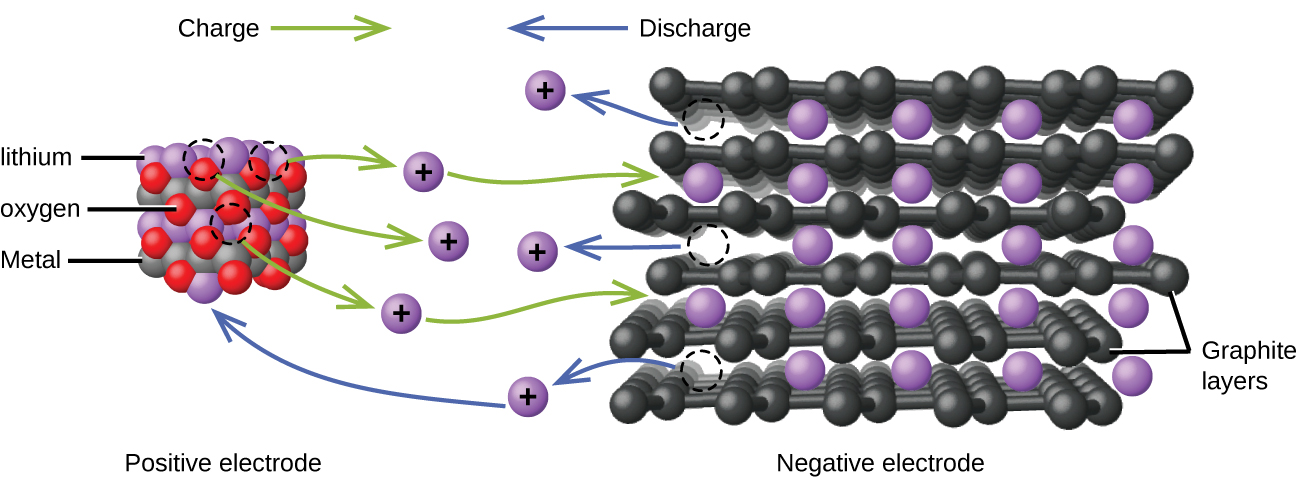Figure 4. In a lithium ion battery, charge flows between the electrodes as the lithium ions move between the anode and cathode.

The lead acid battery (Figure 5) is the type of secondary battery used in your automobile. It is inexpensive and capable of producing the high current required by automobile starter motors. The reactions for a lead acid battery are

$\begin{array}{lr @{{}\longrightarrow{}} l} \text{anode:} & \text{Pb}(s)\;+\;\text{HSO}_4^{\;\;-}(aq) & \text{PbSO}_4(s)\;+\;\text{H}^{+}(aq)\;+\;2\text{e}^{-} \\[0.5em] \text{cathode:} & \text{PbO}_2(s)\;+\;\text{HSO}_4^{\;\;-}(aq)\;+\;3\text{H}^{+}(aq)\;+\;2\text{e}^{-} & \text{PbSO}_4(s)\;+\;2\text{H}_2\text{O}(l) \\[0.5em] \hline \\[-0.25em] \text{overall:} & \text{Pb}(s)\;+\;\text{PbO}_2(s)\;+\;2\text{H}_2\text{SO}_4(aq) & 2\text{PbSO}_4(s)\;+\;2\text{H}_2\text{O}(l) \end{array}$

Each cell produces 2 V, so six cells are connected in series to produce a 12-V car battery. Lead acid batteries are heavy and contain a caustic liquid electrolyte, but are often still the battery of choice because of their high current density. Since these batteries contain a significant amount of lead, they must always be disposed of properly.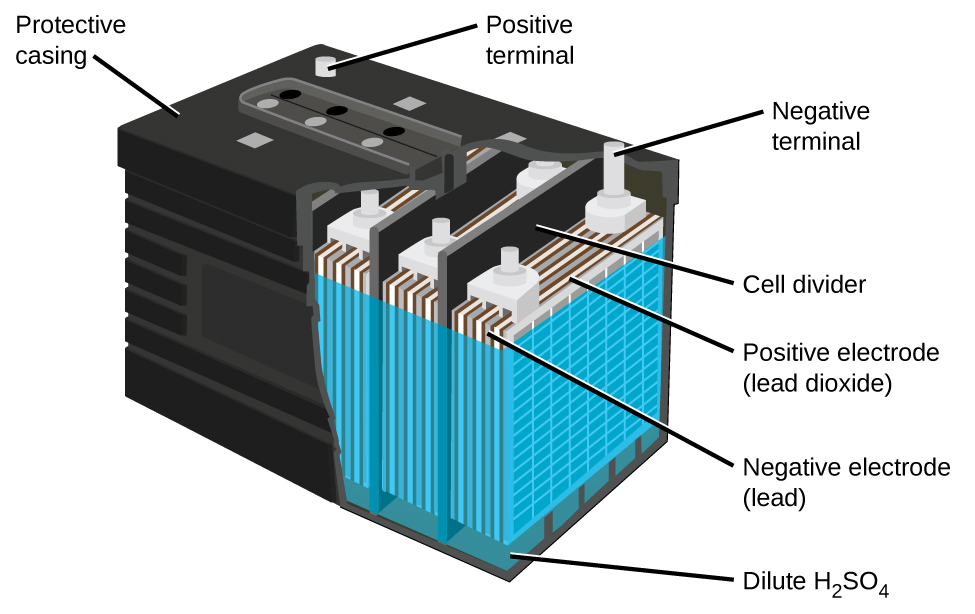Figure 5. The lead acid battery in your automobile consists of six cells connected in series to give 12 V. Their low cost and high current output makes these excellent candidates for providing power for automobile starter motors.

# Fuel Cells

A fuel cell is a device that converts chemical energy into electrical energy. Fuel cells are similar to batteries but require a continuous source of fuel, often hydrogen. They will continue to produce electricity as long as fuel is available. Hydrogen fuel cells have been used to supply power for satellites, space capsules, automobiles, boats, and submarines (Figure 6).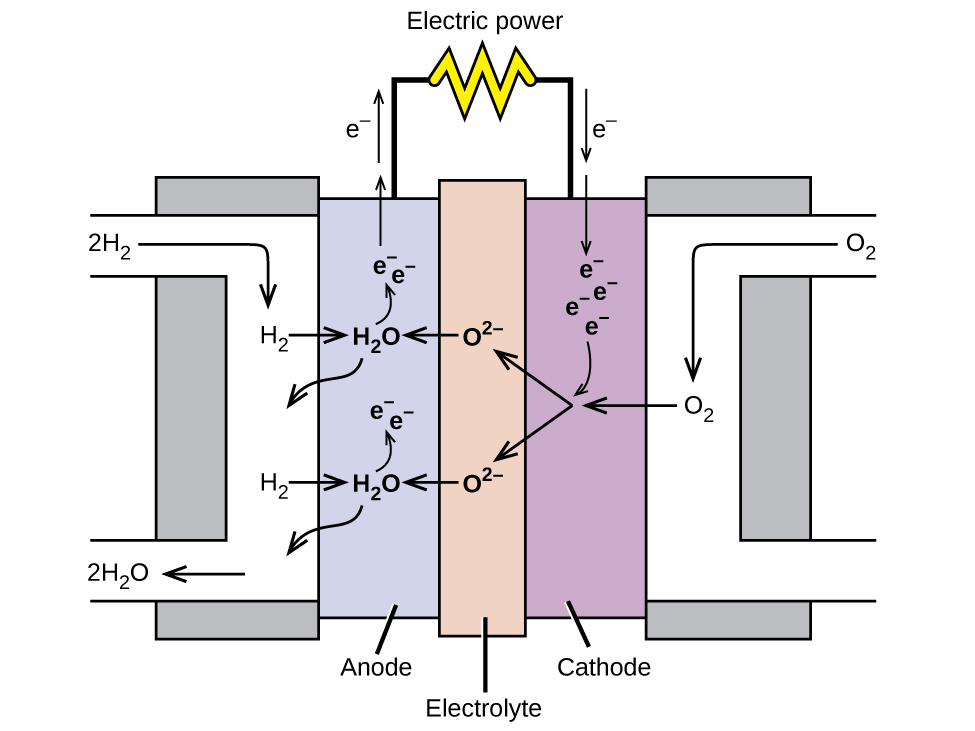Figure 6. In this hydrogen fuel-cell schematic, oxygen from the air reacts with hydrogen, producing water and electricity.

In a hydrogen fuel cell, the reactions are

$\begin{array}{lr @{{}\longrightarrow{}} l} \text{anode:} & 2\text{H}_2\;+\;2\text{O}^{2-} & 2\text{H}_2\text{O}\;+\;4\text{e}^{-} \\[0.5em] \text{cathode:} & \text{O}_2\;+\;4\text{e}^{-} & 2\text{O}^{2-} \\[0.5em] \hline \\[-0.25em] \text{overall:} & 2\text{H}_2\;+\;\text{O}_2 & 2\text{H}_2\text{O} \end{array}$

The voltage is about 0.9 V. The efficiency of fuel cells is typically about 40% to 60%, which is higher than the typical internal combustion engine (25% to 35%) and, in the case of the hydrogen fuel cell, produces only water as exhaust. Currently, fuel cells are rather expensive and contain features that cause them to fail after a relatively short time.

# Key Concepts and Summary

Batteries are galvanic cells, or a series of cells, that produce an electric current. When cells are combined into batteries, the potential of the battery is an integer multiple of the potential of a single cell. There are two basic types of batteries: primary and secondary. Primary batteries are “single use” and cannot be recharged. Dry cells and (most) alkaline batteries are examples of primary batteries. The second type is rechargeable and is called a secondary battery. Examples of secondary batteries include nickel-cadmium (NiCd), lead acid, and lithium ion batteries. Fuel cells are similar to batteries in that they generate an electrical current, but require continuous addition of fuel and oxidizer. The hydrogen fuel cell uses hydrogen and oxygen from the air to produce water, and is generally more efficient than internal combustion engines.

### Chemistry End of Chapter Exercises

1. What are the desirable qualities of an electric battery?
2. List some things that are typically considered when selecting a battery for a new application.
3. Consider a battery made from one half-cell that consists of a copper electrode in 1 M CuSO4 solution and another half-cell that consists of a lead electrode in 1 M Pb(NO3)2 solution.

(a) What are the reactions at the anode, cathode, and the overall reaction?

(b) What is the standard cell potential for the battery?

(c) Most devices designed to use dry-cell batteries can operate between 1.0 and 1.5 V. Could this cell be used to make a battery that could replace a dry-cell battery? Why or why not.

(d) Suppose sulfuric acid is added to the half-cell with the lead electrode and some PbSO4(s) forms. Would the cell potential increase, decrease, or remain the same?

4. Consider a battery with the overall reaction: $\text{Cu}(s)\;+\;2\text{Ag}^{+}(aq)\;{\longrightarrow}\;2\text{Ag}(s)\;+\;\text{Cu}^{2+}(aq)$.

(a) What is the reaction at the anode and cathode?

(b) A battery is “dead” when it has no cell potential. What is the value of Q when this battery is dead?

(c) If a particular dead battery was found to have [Cu2+] = 0.11 M, what was the concentration of silver ion?

5. An inventor proposes using a SHE (standard hydrogen electrode) in a new battery for smartphones that also removes toxic carbon monoxide from the air:
$\begin{array}{lr @{{}\longrightarrow{}} ll} \text{Anode:} & \text{CO}(g)\;+\;\text{H}_2\text{O}(l) & \text{CO}_2(g)\;+\;2\text{H}^{+}(aq)\;+\;2\text{e}^{-} & E_{\text{anode}}^{\circ} = -0.53\;\text{V} \\[0.5em] \text{Cathode:} & 2\text{H}^{+}(aq)\;+\;2\text{e}^{-} & \text{H}_2(g) & E_{\text{cathode}}^{\circ} = 0\;\text{V} \\[0.5em] \hline \\[-0.25em] \text{Overall:} & \text{CO}(g)\;+\;\text{H}_2\text{O}(l) & \text{CO}_2(g)\;+\;\text{H}_2(g) & E_{\text{cell}}^{\circ} = +0.53\;\text{V} \end{array}$

Would this make a good battery for smartphones? Why or why not?

6. Why do batteries go dead, but fuel cells do not?
7. Explain what happens to battery voltage as a battery is used, in terms of the Nernst equation.
8. Using the information thus far in this chapter, explain why battery-powered electronics perform poorly in low temperatures.

## Glossary

alkaline battery
primary battery that uses an alkaline (often potassium hydroxide) electrolyte; designed to be an exact replacement for the dry cell, but with more energy storage and less electrolyte leakage than typical dry cell
battery
galvanic cell or series of cells that produces a current; in theory, any galvanic cell
dry cell
primary battery, also called a zinc-carbon battery; can be used in any orientation because it uses a paste as the electrolyte; tends to leak electrolyte when stored
fuel cell
devices that produce an electrical current as long as fuel and oxidizer are continuously added; more efficient than internal combustion engines
secondary battery that consists of multiple cells; the lead acid battery found in automobiles has six cells and a voltage of 12 V
lithium ion battery
very popular secondary battery; uses lithium ions to conduct current and is light, rechargeable, and produces a nearly constant potential as it discharges
(NiCd battery) secondary battery that uses cadmium, which is a toxic heavy metal; heavier than lithium ion batteries, but with similar performance characteristics
primary battery
single-use nonrechargeable battery
secondary battery
battery that can be recharged

### Solutions

Answers to Chemistry End of Chapter Exercises

2. Considerations include: cost of the materials used in the battery, toxicity of the various components (what constitutes proper disposal), should it be a primary or secondary battery, energy requirements (the “size” of the battery/how long should it last), will a particular battery leak when the new device is used according to directions, and its mass (the total mass of the new device).

4. (a) $\begin{array}{lr @{{}\longrightarrow{}} ll} \text{anode:} & \text{Cu}(s) & \text{Cu}^{2+}(aq)\;+\;2\text{e}^{-} & E_{\text{anode}}^{\circ} = 0.34\;\text{V} \\[0.5em] \text{cathode:} & 2\;\times\;(\text{Ag}^{+}(aq)\;+\;\text{e}^{-} & \text{Ag}(s)) & E_{\text{cathode}}^{\circ} = 0.7996\;\text{V} \end{array}$; (b) 3.5 × 1015; (c) 5.6 × 10−9M

6. Batteries are self-contained and have a limited supply of reagents to expend before going dead. Alternatively, battery reaction byproducts accumulate and interfere with the reaction. Because a fuel cell is constantly resupplied with reactants and products are expelled, it can continue to function as long as reagents are supplied.

8. Ecell, as described in the Nernst equation, has a term that is directly proportional to temperature. At low temperatures, this term is decreased, resulting in a lower cell voltage provided by the battery to the device—the same effect as a battery running dead.